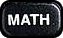## Tech Tips: Counting

### Finding Factorials

To compute $n!$ ...

• R: Assuming $n$ is a value or variable name, use:

factorial(n)


• Excel: Assuming $n$ is a value or cell reference, use:

FACT(n)


• TI-83: Assuming $n$ is a value or variable name, use

n!


The factorial symbol can be typed by pressing the math button and making the following menu selections:: PRB : !

### Finding Combinations

To find ${}_nC_k$, the number of combinations of $n$ things taken $k$ at at time...

• R: Assuming $n$ and $k$ are values or variable names, use:

choose(n,k)


• Excel: Assuming $n$ and $k$ are values or cell references, use:

COMBIN(n,k)


• TI-83: Assuming $n$ and $k$ are values or variable names, use:

n nCr k


The "nCr" above should be typed by pressing the math button and making the following menu selections:: PRB : nCr

### Finding Permutations

To find ${}_nP_k$, the number of permutations of $n$ things taken $k$ at a time...

• R: Assuming $n$ and $k$ are values or variable names, and recalling that $\displaystyle{{}_n P_k = k! \cdot ({}_n C_k)}$ use:

factorial(k)*choose(n,k)


• Excel: Assuming $n$ and $k$ are values or cell references, use:

PERMUT(n,k)


• TI-83: Assuming $n$ and $k$ are values or variable names, use:

n nPr k


The "nPr" above should be typed by pressing the math button and making the following menu selections:: PRB : nPr

### Finding Permutations with Identical Elements

To find the number of ways to arrange $n$ objects when there are $k_1$ identical items of one type, $k_2$ identical items of a second type, $k_3$ identical items of a third type, etc. (where $k_1 + k_2 + k_3 + \cdots = n$) ...

• R: Assuming $k_1$, $k_2$, $k_3$, etc. are values or variable names, and recalling that the number we seek is given by $$\frac{n!}{k_1! \cdot k_2! \cdot k_3! \cdots}$$ the most convenient approach is to store these $k_i$ values into a vector first, and then perform the calculation, as shown below:

counts = c(k1,k2,k3,...)
factorial(sum(counts))/prod(factorial(counts))


As an example, the number of ways to arrange the letters of the word "MISSISSIPPI", given that there is 1 "M", 4 "I"'s, 4 "S"'s, and 2 "P"'s can be calculated with

> counts = c(1,4,4,2)
> factorial(sum(counts))/prod(factorial(counts))
 34650


One should be careful not to neglect the items that only appear once in the vector - as otherwise the numerator of the fraction calculated will be an incorrect sum.

• Excel: Assuming $k_1$, $k_2$, $k_3$, etc. are values or cell references, use:

MULTINOMIAL(k1,k2,k3,...)

To redo the example above in the context of Excel, the following finds the number of ways to arrange the letters of the word "MISSISSIPPI":
MULTINOMIAL(1,4,4,2)

(Just as the equivalent expression did in R above, this expression evaluates to 34650.)

• TI-83: Assuming $k_1$, $k_2$, $k_3$, etc. are values or variable names, and again recalling that the number we seek is given by $$\frac{n!}{k_1! \cdot k_2! \cdot k_3! \cdots}$$ we simply use:

(k1+k2+k3+...)!/(k1!*k2!*k3!*...)

or if $n = k_1 + k_2 + k_3 + \cdots$ is known, the following is of course simpler:
n!/(k1!*k2!*k3!*...)

For the "MISSISSIPPI" example above, one would have
11!/(4!*4!*2!)

This too, evaluates to 34650.

Be careful to always include the parentheses around the factors of the denominator, as the order of operations will have you multiplying things by all but the first of these factors otherwise.